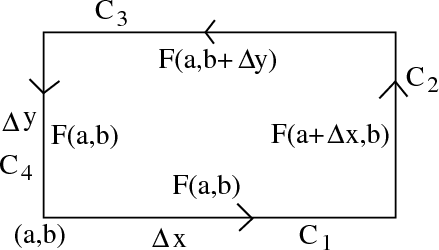# Math Insight

### Image: The vector field approximation used to derive a formula for the circulation per unit areaThe vector field $\dlvf$ is approximated to be constant on each side of the rectangular curve $\dlc$ in a step of the derivation of the circulation per unit area formula.

Image file: circulation_unit_area_calculation_field.png